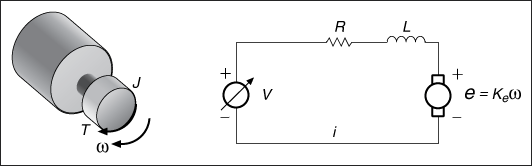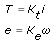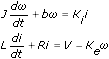# Part 1: Choosing a Proper Dynamic System Model by Using Physical Modeling (System Identification Toolkit)

LabVIEW 2013 System Identification Toolkit Help

Edition Date: June 2013

Part Number: 372458D-01

»View Product InfoDownload Help (Windows Only)

Before you estimate a dynamic system, create a mathematical model that contains unknown coefficients. You then use system identification to estimate these coefficients.

Physical modeling is a common method for sketching a mathematical model that represents a real dynamic system. Physical modeling determines the nature of the model, such as the model order and the number of I/O channels. Use physical modeling to define proper models for dynamic systems.

The following figure shows an electrical circuit model of a brushed direct current (DC) servomotor.where V is the source voltage of the DC power supply. R is the resistance of the DC servomotor armature circuit. L is the inductance of the DC servomotor armature circuit. i is the circuit armature current. ω is the shaft speed of the DC servomotor. T is the torque of the DC servomotor.

In this dynamic system, the source voltage, V, is the input and the DC servomotor shaft speed, ω, is the output.

According to Faraday's Law of electromagnetic induction, the circuit armature current i, motor torque T, motor shaft velocity ω, and motor back-EMF voltage e, have the following relationship:where Kt is an electromotive force constant. Ke is a motor back-EMF constant.

You can obtain the following equations by using Newton's Law and Kirchhoff's Law.where J is the moment of inertia of the rotor. b is the damping ratio of the mechanical part of the DC servomotor.

Perform further transforms on these equations to obtain a mathematic model of the dynamic system. You can choose one of the four common dynamic system models on the basis of your needs.Note  If you perform system identification with an improper or incorrect physical model, the resulting dynamic system model may not correctly describe the behaviors of the dynamic system.